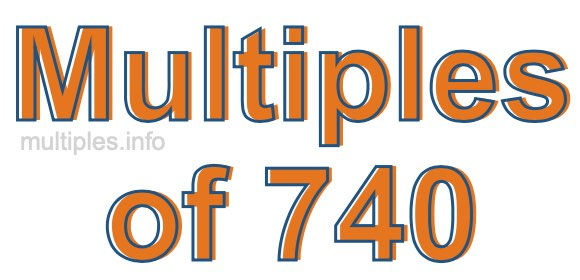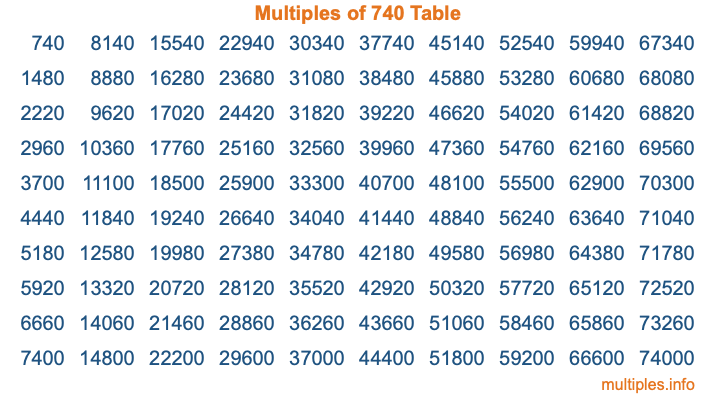Multiples of 740Welcome to the Multiples of 740 page. Here we will first teach you everything you will ever need to know about the multiples of 740, and then give you a study guide summary of everything we taught you to make sure you remember it all. Use this page to look up facts and learn information about the multiples of 740. This page will make you a multiples of seven hundred forty expert!

Definition of Multiples of 740
Multiples of 740 are all the numbers that when divided by 740 equal an integer. Each of the multiples of 740 are called a multiple. A multiple of 740 is created by multiplying 740 by an integer.

Therefore, to create a list of multiples of 740, you start with 1 multiplied by 740, then 2 multiplied by 740, then 3 multiplied by 740, and so on for as long as you want. Thus, the list of the first five multiples of 740 is 740, 1480, 2220, 2960, and 3700. To see a larger list of multiples of 740, see the printable image of Multiples of 740 further down on this page. We also have a category where you can choose any nth multiple of 740.

Multiples of 740 Checker
The Multiples of 740 Checker below checks to see if any number of your choice is a multiple of 740. In other words, it checks to see if there is any number (integer) that when multiplied by 740 will equal your number. To do that, we divide your number by 740. If the the quotient is an integer, then your number is a multiple of 740.

Is  a multiple of 740?

Least Common Multiple of 740 and ...
A Least Common Multiple (LCM) is the lowest multiple that two or more numbers have in common. This is also called the smallest common multiple or lowest common multiple and is useful to know when you are adding our subtracting fractions. Enter one or more numbers below (740 is already entered) to find the LCM.

Check out our LCM Calculator if you need more details about the Least Common Multiple or if you need the LCM for different numbers for adding and subtraction fractions.

nth Multiple of 740
As we stated above, 740 is the first multiple of 740, 1480 is the second multiple of 740, 2220 is the third multiple of 740, and so on. Enter a number below to find the nth multiple of 740.

th multiple of 740

Multiples of 740 vs Factors of 740
740 is a multiple of 740 and a factor of 740, but that is where the similarities end. All postive multiples of 740 are 740 or greater than 740. All positive factors of 740 are 740 or less than 740.

Below is the beginning list of multiples of 740 and the factors of 740 so you can compare:

Multiples of 740: 740, 1480, 2220, 2960, 3700, etc.

Factors of 740: 1, 2, 4, 5, 10, 20, 37, 74, 148, 185, 370, 740

As you can see, the multiples of 740 are all the numbers that you can divide by 740 to get a whole number. The factors of 740, on the other hand, are all the whole numbers that you can multiply by another whole number to get 740.

It's also interesting to note that if a number (x) is a factor of 740, then 740 will also be a multiple of that number (x).

Multiples of 740 vs Divisors of 740
The divisors of 740 are all the integers that 740 can be divided by evenly. Below is a list of the divisors of 740.

Divisors of 740: 1, 2, 4, 5, 10, 20, 37, 74, 148, 185, 370, 740

The interesting thing to note here is that if you take any multiple of 740 and divide it by a divisor of 740, you will see that the quotient is an integer.

Multiples of 740 Table
Below is an image of the first 100 multiples of 740 in a table. The table is in chronological order, column by column. The first column has the first ten multiples of 740, the second column has the next ten multiples of 740, and so on.The Multiples of 740 Table is also referred to as the 740 Times Table or Times Table of 740. You are welcome to print out our table for your studies.

Negative Multiples of 740
Although not often discussed or needed in math, it is worth mentioning that you can make a list of negative multiples of 740 by multiplying 740 by -1, then by -2, then by -3, and so on, to get the following list of negative multiples of 740:

-740, -1480, -2220, -2960, -3700, etc.

Multiples of 740 Summary
Below is a summary of important Multiples of 740 facts that we have discussed on this page. To retain the knowledge on this page, we recommend that you read through the summary and explain to yourself or a study partner why they hold true.

There are an infinite number of multiples of 740.

A multiple of 740 divided by 740 will equal a whole number.

740 divided by a factor of 740 equals a divisor of 740.

The nth multiple of 740 is n times 740.

The largest factor of 740 is equal to the first positive multiple of 740.

740 is a multiple of every factor of 740.

740 is a multiple of 740.

A multiple of 740 divided by a divisor of 740 equals an integer.

740 divided by a divisor of 740 equals a factor of 740.

Any integer times 740 will equal a multiple of 740.

Multiples of a Number
Here you can get the multiples of another number, all with the same attention to detail as we did for multiples of 740 on this page.

Multiples of
Multiples of 741
Did you find our page about multiples of seven hundred forty educational? Do you want more knowledge? Check out the multiples of the next number on our list!